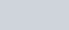# Spring potential energy | definition, meaning and its derivation

• 16
•
• 2
•
•
•
•
•
18
Shares

We that know how potential energy is defined for any conservative forces, here we will derive the Potential energy of spring. All the results are derived assuming ideal springs.

# The potential energy of Spring

### Meaning of potential energy of spring

We can see that if we compress or elongate a spring, we feel opposing force by spring, Whenever we disturb spring from the natural length it tries to retain its natural length, therefore there is some energy stored in a compressed or stretched spring due to which it tries to retain natural length.

Actually, this energy stored in any stretched/compressed spring or in stretching of any rubber band, etc, is elastic potential energy as the bodies are resisting change in its shape, size, length, etc.

### Derivation of the potential energy of spring

#### When compressing a spring from the natural length

Spring force is conservative therefore by conservative field theory we know that

(1)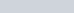Suppose we have a spring of natural length “l”. We compress it by x length from the natural length, now in this compressed state spring is capable of doing work hence having energy.

Now work done by spring in compressing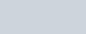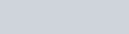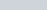Because force and displacement are opposite.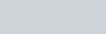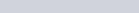(2)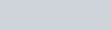From equation (1) and (2)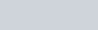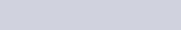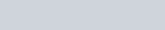If we take x = 0 i.e. natural length and take it as a reference point and assume the potential energy at natural length to be zero, then

(3)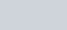compress a spring by an amount “x” w.r.t natural length the potential energy stored in spring at that state is given by equation (3).

#### When stretching a spring from natural length.

We know that whether we stretch or compress a spring by “x” amount from the natural length the work done by spring in both cases is the same.

Therefore if we strech spring by “x” amount.

(4)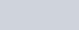From equation (1) and (4) .If we take x = 0 i.e. natural length and take it as a reference point and assume the potential energy at natural length to be zero, then

(5)Stretch a spring by an amount “x” w.r.t natural length the potential energy stored in spring at that state is given by equation (5).

# Result

From equations (3) and (5), we can see that whether we compress or stretch a spring by an amount “x” the amount of potential energy of spring in that state is the same in both springs.

Given by

(6)